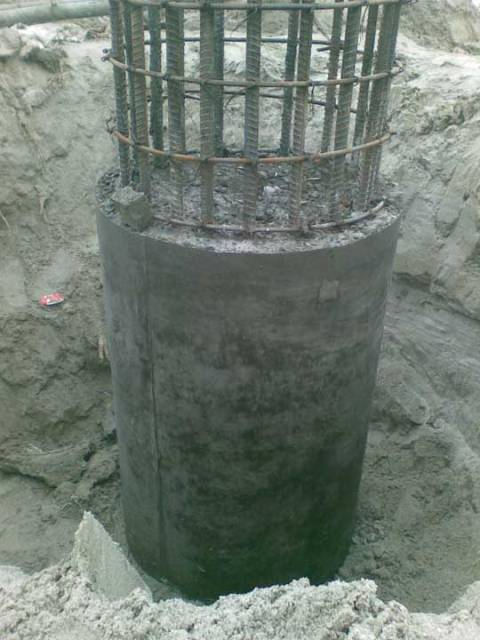# HOW TO CALCULATE PILE LOAD CAPACITY IN COHESIONLESS SOIL USING SPT OR N-VALUES?

## Pile Load Capacity Calculation Using Standard Penetration Resistance

This method of calculating pile load capacity in cohesion less soil (i.e. in sand) was suggested by Meyerhof.

Meyerhof suggested the following equation, to calculate the ultimate pile load capacity in saturated sand, using standard penetration resistance (N). In the formula given below the 1st term gives the end bearing resistance of pile and the 2nd term gives the skin friction resistance of pile.Where,

Qu = Ultimate load capacity of pile, in kN

N = Average ‘N’ value at pile tip

L = Length of pile embedded in soil, in m

B = Diameter or width of pile, in m

Ap = Cross-sectional area of pile tip, in m2

N’ = Average ‘N’ along the pile shaft

As = Surface area of pile shaft

#### Notes to remember

1. In no case the end bearing resistance (i.e. the 1st term) should be more than 130NAp.
2. For non-plastic silt or very fine sand the above formula should be replaced by the following formula.3. The safe pile load capacity (Qs) can be obtained by dividing a suitable factor of safety by ultimate load capacity (Qu) as calculated above, i.e.

Qs = Qu / F.O.S.

The minimum value of factor of safety (F.O.S.) is taken as 2.5. But it should be remembered that the value of F.O.S. is dependent upon the following three factors.

• Reliability of the formula
• Reliability of the sub soil parameter
• Allowable settlement of the structure